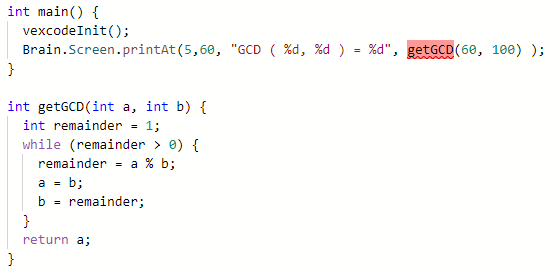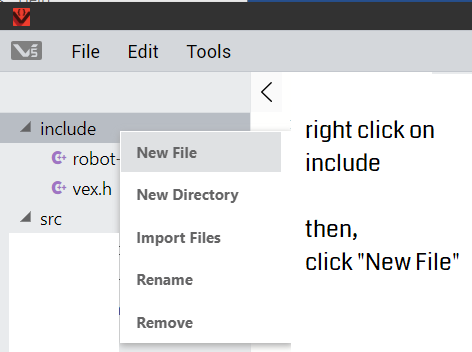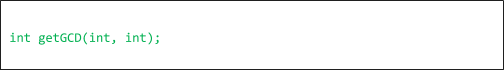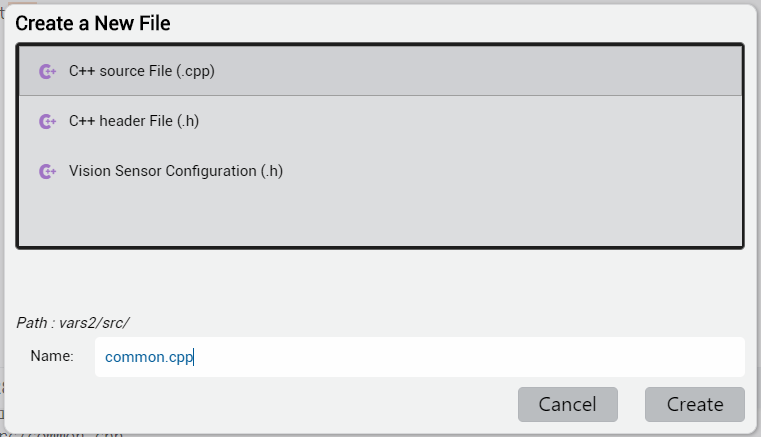# Understanding the Syntax to Create Functions in VEXcode Pro V5

## Function definition

Now that we have gone through a few samples, let’s look at the general form of a function definition:

```void function-name() {

Declarations of variables, etc.
Expressions...
//no explicit return is necessary.
}
```

A function returns no special value, nor no incoming parameters.

```void function-name(data-type variable) {

Declarations of variables, etc.
Expressions...
//no explicit return is necessary.
}

If you want multiple variables, you simply use "," as a delimitor:
```

A function returns no special value, but with one parameter.

```void function-name(data-type variable, data-type variable, etc.) {

Declarations of variables, etc.
Expressions...
//no explicit return is necessary.
}
```

A function returns no special value, but with more than one parameter.

```void function-name(data-type variable, data-type variable, etc.) {

Declarations of variables, etc.
Expressions...
return return-value
}
```

A function returns a value of a specific datatype, and with more than input one parameter.

The return type of a function must be the same as the data type of the return-value that function returns. The return-value can be a constant value or a variable. It must be preceded with the keyword “return.

The following rules govern the return type:

• There are almost no restrictions on the return type, except array. (Array is another more advanced topic regarding how to create your data. It is outside the scope of this article and not be covered here.
• Specifying that the return type “void” means no return value is necessary. The clause “return” is implicit. That means you have to explicitly state “return” at the end of a “void” function.

## Creating a function before the main() or after?

The compiler reads your file from top to bottom. Thus, the order does matter.

Let’s take the simple Greatest Common Denominator (GCD) function as an example. You will get an error: `use of undeclared identifier 'getGCD'`Actually this is true whether it is from the main( ) or other calling function block, i.e. the “called function block” must precede the “calling function block.”

Two possible solutions:

```int getGCD(int a, int b) {
int remainder = 1;
while (remainder > 0) {
remainder = a % b;
a = b;
b = remainder;
}
return a;
}

int main() {
Brain.Screen.printAt(5,60, “GCD ( %d, %d ) = %d”, getGCD(60, 100) );
}
```

Move the “called function” above the “calling function block,” in the example "main()."

```int getGCD(int, int);

int main() {
Brain.Screen.printAt(5,60, "GCD ( %d, %d ) = %d", getGCD(60, 100) );
}

int getGCD(int a, int b) {
int remainder = 1;
while (remainder > 0) {
remainder = a % b;
a = b;
b = remainder;
}
return a;
}
```

Put the prototype (also called signature) of the function before the “calling function block.”

## What if you wish to modularize functions in different files?

##### Step 1: Create a header file, and put the prototype of the function into this file.

e.g. create a file named “myFuncs.h”

You need to “add” this header file into your project before compiling your code. This allows the VEXcode Pro V5 IDE “to be aware of” the inclusion of this new header file before it starts building your project.

Here is how:In this header file “common.h” (you can name whatever you want, as long as it is alphanumeric with no space.)

##### Step 2: Create a separate cpp file, e.g., called common.cpp```//this is the common.cpp file

int getGCD(int a, int b) {
int remainder = 1;
while (remainder > 0) {
remainder = a % b;
a = b;
b = remainder;
}
return a;
}
```

Move the function getGCD(...) into this file

```#include "vex.h"
#include "common.h"
using namespace vex;

int main() {
vexcodeInit();
Brain.Screen.printAt(5,60, "GCD ( %d, %d ) = %d", getGCD(60, 100) );
}
```

Now, all you have in main cpp file will consist of the following

Future topics will be covered in more advanced sections of the VEX Library in the future:

• Pass by value vs. pass by reference
• Pass in an array
• Pass in a structure

For more information, help, and tips, check out the many resources at VEX Professional Development Plus

Last Updated: